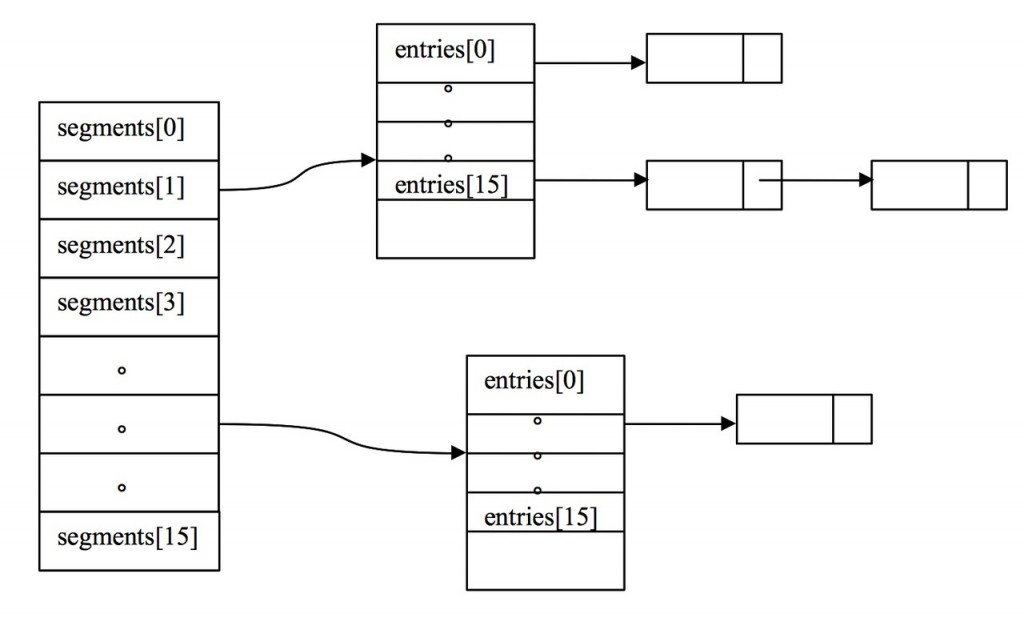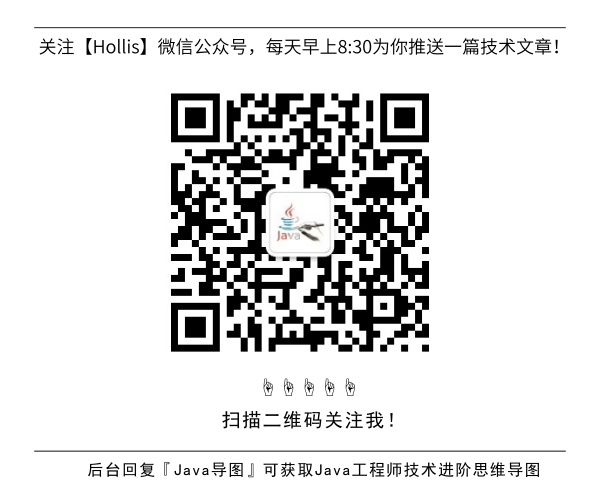# HashMap完全解读

GitHub 19k Star 的Java工程师成神之路，不来了解一下吗！

### HashMap和HashTable的区别

1.HashTable的方法是同步的，在方法的前面都有synchronized来同步，HashMap未经同步，所以在多线程场合要手动同步
2.HashTable不允许null值(key和value都不可以) ,HashMap允许null值(key和value都可以)。
3.HashTable有一个contains(Object value)功能和containsValue(Object value)功能一样。
4.HashTable使用Enumeration进行遍历，HashMap使用Iterator进行遍历。
5.HashTable中hash数组默认大小是11，增加的方式是 old*2+1。HashMap中hash数组的默认大小是16，而且一定是2的指数。
6.哈希值的使用不同，HashTable直接使用对象的hashCode，代码是这样的：

``````int hash = key.hashCode();
int index = (hash & 0x7FFFFFFF) % tab.length;
``````

``````int hash = hash(k);
int i = indexFor(hash, table.length);
static int hash(Object x) {
h ^= (h >>> 20) ^ (h >>> 12);
return h ^ (h >>> 7) ^ (h >>> 4);
}
static int indexFor(int h, int length) {
return h & (length-1);
}
``````

### 三、HashMap与HashSet的关系

1、HashSet底层是采用HashMap实现的：

public HashSet() {
map = new HashMap<E,Object>();
}

``````private static final Object PRESENT = new Object(); public boolean add(E e) {
return map.put(e, PRESENT)==null;
}
public boolean remove(Object o) {
return map.remove(o)==PRESENT;
}
``````

### 五、HashMap实现原理分析

1. HashMap的数据结构 数据结构中有`数组``链表`来实现对数据的存储，但这两者基本上是两个极端。

哈希表有多种不同的实现方法，我接下来解释的是最常用的一种方法—— 拉链法，我们可以理解为“链表的数组” ，如图：从上图我们可以发现哈希表是由数组+链表组成的，一个长度为16的数组中，每个元素存储的是一个链表的头结点。那么这些元素是按照什么样的规则存储到数组中呢。一般情况是通过hash(key)%len获得，也就是元素的key的哈希值对数组长度取模得到。比如上述哈希表中，12%16=12,28%16=12,108%16=12,140%16=12。所以12、28、108以及140都存储在数组下标为12的位置。 　　HashMap其实也是一个线性的数组实现的,所以可以理解为其存储数据的容器就是一个线性数组。这可能让我们很不解，一个线性的数组怎么实现按键值对来存取数据呢？这里HashMap有做一些处理。 　　首先HashMap里面实现一个静态内部类Entry，其重要的属性有 key , value, next，从属性key,value我们就能很明显的看出来Entry就是HashMap键值对实现的一个基础bean，我们上面说到HashMap的基础就是一个线性数组，这个数组就是Entry[]，Map里面的内容都保存在Entry[]里面。

### 六、HashMap的存取实现

``````// 存储时:
int hash = key.hashCode(); // 这个hashCode方法这里不详述,只要理解每个key的hash是一个固定的int值
int index = hash % Entry[].length;
Entry[index] = value;

// 取值时:
int hash = key.hashCode();
int index = hash % Entry[].length;
return Entry[index];
``````

1）put

``````public V put(K key, V value) {
if (key == null)
return putForNullKey(value); //null总是放在数组的第一个链表中
int hash = hash(key.hashCode());
int i = indexFor(hash, table.length);
//遍历链表
for (Entry<K,V> e = table[i]; e != null; e = e.next) {
Object k;
//如果key在链表中已存在，则替换为新value
if (e.hash == hash && ((k = e.key) == key || key.equals(k))) {
V oldValue = e.value;
e.value = value;
e.recordAccess(this);
return oldValue;
}
}
modCount++;
addEntry(hash, key, value, i);
return null;
}

void addEntry(int hash, K key, V value, int bucketIndex) {
Entry<K,V> e = table[bucketIndex];
table[bucketIndex] = new Entry<K,V>(hash, key, value, e); //参数e, 是Entry.next
//如果size超过threshold，则扩充table大小。再散列
if (size++ >= threshold)
resize(2 * table.length);
}
``````

2）get

``````public V get(Object key) {
if (key == null)
return getForNullKey();
int hash = hash(key.hashCode());
//先定位到数组元素，再遍历该元素处的链表
for (Entry<K,V> e = table[indexFor(hash, table.length)];
e != null;
e = e.next) {
Object k;
if (e.hash == hash && ((k = e.key) == key || key.equals(k)))
return e.value;
}
return null;
}
``````

3）null key的存取

null key总是存放在Entry[]数组的第一个元素。

``````   private V putForNullKey(V value) {
for (Entry<K,V> e = table; e != null; e = e.next) {
if (e.key == null) {
V oldValue = e.value;
e.value = value;
e.recordAccess(this);
return oldValue;
}
}
modCount++;
addEntry(0, null, value, 0);
return null;
}

private V getForNullKey() {
for (Entry<K,V> e = table; e != null; e = e.next) {
if (e.key == null)
return e.value;
}
return null;
}
``````

4）确定数组index：hashcode % table.length取模

HashMap存取时，都需要计算当前key应该对应Entry[]数组哪个元素，即计算数组下标；算法如下：

`````` /**
* Returns index for hash code h.
*/
static int indexFor(int h, int length) {
return h & (length-1);
}
``````

5）table初始大小

``````public HashMap(int initialCapacity, float loadFactor) {
.....
// Find a power of 2 >= initialCapacity
int capacity = 1;
while (capacity < initialCapacity)
capacity <<= 1;
threshold = (int)(capacity * loadFactor);
table = new Entry[capacity];
init();
}
``````

### 八、 再散列rehash过程

`````` /**
* Rehashes the contents of this map into a new array with a
* larger capacity.  This method is called automatically when the
* number of keys in this map reaches its threshold.
*
* If current capacity is MAXIMUM_CAPACITY, this method does not
* resize the map, but sets threshold to Integer.MAX_VALUE.
* This has the effect of preventing future calls.
*
* @param newCapacity the new capacity, MUST be a power of two;
*        must be greater than current capacity unless current
*        capacity is MAXIMUM_CAPACITY (in which case value
*        is irrelevant).
*/
void resize(int newCapacity) {
Entry[] oldTable = table;
int oldCapacity = oldTable.length;
if (oldCapacity == MAXIMUM_CAPACITY) {
threshold = Integer.MAX_VALUE;
return;
}
Entry[] newTable = new Entry[newCapacity];
transfer(newTable);
table = newTable;
threshold = (int)(newCapacity * loadFactor);
}

/**
* Transfers all entries from current table to newTable.
*/
void transfer(Entry[] newTable) {
Entry[] src = table;
int newCapacity = newTable.length;
for (int j = 0; j < src.length; j++) {
Entry<K,V> e = src[j];
if (e != null) {
src[j] = null;
do {
Entry<K,V> next = e.next;
//重新计算index
int i = indexFor(e.hash, newCapacity);
e.next = newTable[i];
newTable[i] = e;
e = next;
} while (e != null);
}
}
}
``````
(全文完)### 评论 4

1. #1

好[/强]

听雨小憩7年前 (2016-03-05)回复
2. #2

数组的二分查找时间复杂度小，为O(1)好像不太对吧！！
因为计算机能够获得该数组的首地址，从而获得arr[i]就没有复杂度了！！【数组元素所占的字节数是一定的，且数组是在一个连续的内存中存储】

一横三竖7年前 (2016-03-22)回复
• 你好，表述确实有问题。
二分查找每次把搜索区域减少一半，所以时间复杂度应该是O(log n)。
数组利用下标定位元素，时间复杂度应该为O(1)。

HollisChuang7年前 (2016-03-22)回复
3. #3

hashmap的数据结构图错了 那个是concurrenthashmap的

小明6号12245年前 (2017-09-24)回复

## HollisChuang's Blog

• 微信咨询
• 去评论
• 回顶
###### 回顶部